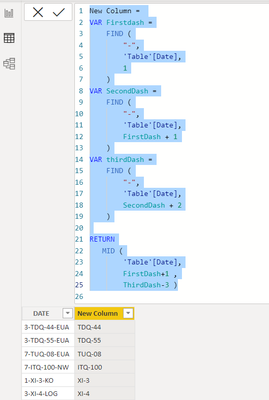cancel
Showing results for
Did you mean:Helper I

## CHALLENGE CALCULATED COLUMN

I have this table :

 DATE RESULT 3-TDQ-44-EUA TDQ-44 3-TDQ-55-EUA TDQ-55 7-TUQ-08-EUA TUQ-08 7-ITQ-100-NW ITQ-100 1-XI-3-KO XI-3 3-XI-4-LOG XI-4 3-XI-7-KO XI-7 7-XI-10-EBG XI-10 7-XI-11D-KO XI-11D 4-XI-12-ERL XI-12 IC-1- IC-1 IC-6 IC-6

I would like to extract the date information to get the data as resulting using DAX is it possible?

1 ACCEPTED SOLUTIONSuper User

``````New Column =
VAR _Len =
LEN ( 'Table'[DATE] )
VAR _Dash_No =
_Len - LEN ( SUBSTITUTE ( 'Table'[DATE], "-", "" ) )
VAR _C =
IF ( LEFT ( RIGHT ( 'Table'[DATE], 4 ), 1 ) = "-", 4, 3 )
VAR _K =
IF ( _Dash_No > 2, RIGHT ( 'Table'[DATE], _Len - 2 ), 'Table'[DATE] )
VAR _Re =
IF ( _Dash_No > 2, LEFT ( _K, LEN ( _K ) - _C ), LEFT ( 'Table'[DATE], 4 ) )
RETURN
_Re``````

Output:If this post helps, please consider accepting it as the solution to help the other members find it more quickly.6 REPLIES 6Helper I

It turned out wonderful. I need to study the lines point by point to try to make some adaptations. and see if it works.Super User

Hi:

You can try this calculatedcolmun:

New Column =
VAR Firstdash =
FIND (
"-",
'Table'[Date],
1
)
VAR SecondDash =
FIND (
"-",
'Table'[Date],
FirstDash + 1
)
VAR thirdDash =
FIND (
"-",
'Table'[Date],
SecondDash + 2
)

RETURN
MID (
'Table'[Date],
FirstDash+1 ,
ThirdDash-3 )
I hope this is a good solution for you.Helper I

I tried to use FIND but this error appears "Could not find the Search text given to function 'FIND' in the text in question."Super User

Hi:

That is strange. Please see attached solution. The message is saying what ever you have between the " " it couldn't find. I copied your data and it's the dash it is finding. "-" . If you put an extra space or did not use - then it can't find it. Can you try again as the DAX is the most easy to understand for future applications for you and community.

Please mark as solution if you may having been putting an extra space between "-". Or using _ instead of -. I hope this solution makes sense. Thanks

New Column =
VAR Firstdash =
FIND (
"-",
'Table'[Date],
1
)
VAR SecondDash =
FIND (
"-",
'Table'[Date],
FirstDash + 1
)
VAR thirdDash =
FIND (
"-",
'Table'[Date],
SecondDash + 2
)

RETURN
MID (
'Table'[Date],
FirstDash+1 ,
ThirdDash-3 )Super User

``````New Column =
VAR _Len =
LEN ( 'Table'[DATE] )
VAR _Dash_No =
_Len - LEN ( SUBSTITUTE ( 'Table'[DATE], "-", "" ) )
VAR _C =
IF ( LEFT ( RIGHT ( 'Table'[DATE], 4 ), 1 ) = "-", 4, 3 )
VAR _K =
IF ( _Dash_No > 2, RIGHT ( 'Table'[DATE], _Len - 2 ), 'Table'[DATE] )
VAR _Re =
IF ( _Dash_No > 2, LEFT ( _K, LEN ( _K ) - _C ), LEFT ( 'Table'[DATE], 4 ) )
RETURN
_Re``````

Output:If this post helps, please consider accepting it as the solution to help the other members find it more quickly.Helper I
 RESULT TEXT XI-7 TICKET 3-XI-7-KO XI-10 TICKET 7-XI-10-EBG XI-11D TICKET 7-XI-11D-KO XI-12 TICKET 4-XI-12-ERL IC-1 TICKET IC-1- IC-6 TICKET IC-6 EU-226 TICKET 7-EU-226-SW FLK-86IN TICKET 7-FLK-86IN-GU Glove CHIEN KLOW HH

Is there any simpler way to get it straight from the text?